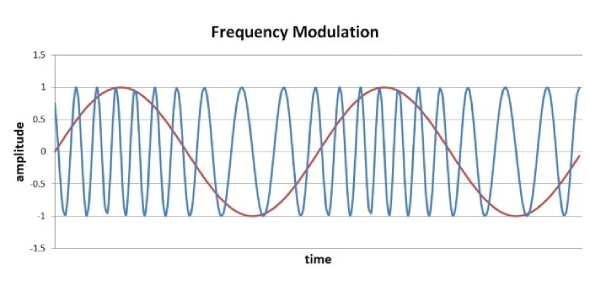Frenzel Chapter 4: Frequency Modulation

29 Questions | Total Attempts: 2935SettingsThis quiz is created by the people behind www. Ecesources. Blogspot. Com

• 1.
The amount of frequency deviation from the carrier center frequency in an FM transmitter is proportional to what characteristic of the modulating signal?
• A.

Amplitude

• B.

Frequency

• C.

Phase

• D.

Shape

• 2.
Both FM and PM are types of what kind of modulation?
• A.

Amplitude

• B.

Phase

• C.

Angle

• D.

Duty Cycle

• 3.
If the amplitude of the modulating signal decreases, the carrier deviation
• A.

Increases

• B.

Decreases

• C.

Remains constant

• D.

Goes to zero

• 4.
On an FM signal, maximum deviation occurs at what point on the modulating signal?
• A.

Zero crossing points

• B.

Peak positive amplitude

• C.

Peak negative amplitude

• D.

Both B and C

• 5.
In PM, a frequency shift occurs while what characteristic of the modulating signal is changing?
• A.

Shape

• B.

Phase

• C.

Frequency

• D.

Amplitude

• 6.
Maximum frequency deviation of a PM signal occurs at
• A.

Zero crossing points

• B.

Peak positive amplitude

• C.

Peak negative amplitude

• D.

Peak positive or negative amplitude

• 7.
In PM, carrier frequency deviation is not proportional to
• A.

Modulating signal amplitude

• B.

Carrier amplitude and frequency

• C.

Modulating signal frequency

• D.

Modulator phase shift

• 8.
To compensate for increases in carrier frequency deviation with an increase in modulating signal frequency, what circuit is used between the modulating signal and the phase modulator?
• A.

Low pass filter

• B.

High pass filter

• C.

Phase shifter

• D.

Bandpass filter

• 9.
The FM produced by PM is called
• A.

FM

• B.

PM

• C.

Indirect FM

• D.

Indirect PM

• 10.
If the amplitude of the modulating signal applied to a phase modulator is constant, the output signal will be
• A.

Zero

• B.

The carrier frequency

• C.

Above the carrier frequency

• D.

Below the carrier frequency

• 11.
A 100 MHz carrier is deviated 50 KHz by a 4KHz signal. The modulation index is
• A.

5

• B.

8

• C.

12.5

• D.

20

• 12.
The maximum deviation of an FM carrier is 2KHz by a maximum modulating signal of 400Hz. The deviation ratio is
• A.

0.2

• B.

5

• C.

8

• D.

40

• 13.
A 70 KHz carrier has a frequency deviation of 4KHz with a 1000Hz signal. How many significant sideband pairs are produced?
• A.

4

• B.

5

• C.

6

• D.

7

• 14.
What is the relative amplitude of the third pair of sidebands of an FM signal with m=6?
• A.

0.11

• B.

0.17

• C.

0.24

• D.

0.36

• 15.
A 200KHz carrier is modulated by a 2.5KHz signal. The fourth pair of sidebands are spaced from the carrier by
• A.

2.5KHz

• B.

5KHz

• C.

10KHz

• D.

15KHz

• 16.
An FM transmitter has a maximum deviation of 12 KHz and a maxium modulating frequency of 12 KHz. The bandwidth by Carson's rule is
• A.

24KHz

• B.

33.6KHz

• C.

36.8KHz

• D.

48KHz

• 17.
The maximum allowed deviation of the FM sound signal in TV is 25KHz. If the actual deviation is 18KHz, the percent modulation is
• A.

43 percent

• B.

72 percent

• C.

96 percent

• D.

139 percent

• 18.
Which of the following is not a major benefit of FM over AM?
• A.

Greater efficiency

• B.

Noise immunity

• C.

Capture effect

• D.

Lower complexity and cost

• 19.
The primary disadvantage of FM is its
• A.

Higher cost and complexity

• B.

Excessive use of spectrum space

• C.

Noise susceptibility

• D.

Lower efficiency

• 20.
Noise is primarily
• A.

High frequency spikes

• B.

Low frequency variations

• C.

Random level shifts

• D.

Random frequency variations

• 21.
The receiver circuit that rids FM of noise is the
• A.

Modulator

• B.

Demodulator

• C.

Limiter

• D.

Low pass filter

• 22.
The phenomenon of a strong FM signal dominating a weaker signal on a common frequency is referred to as the
• A.

Capture effect

• B.

Blot out

• C.

Quieting factor

• D.

Domination syndrome

• 23.
The AM signals generated at a low level may only be amplified by what type of amplifier?
• A.

Op Amp

• B.

Linear

• C.

Class C

• D.

Push-pull

• 24.
Frequency modulation transmitters are more efficient because their power is increased by what type of amplifier?
• A.

Class A

• B.

Class B

• C.

Class C

• D.

All of the above

• 25.
Noise interfere mainly with modulating signals that are
• A.

Sinusoidal

• B.

Nonsinusoidal

• C.

Low frequencies

• D.

High frequencies

Related TopicsBack to top